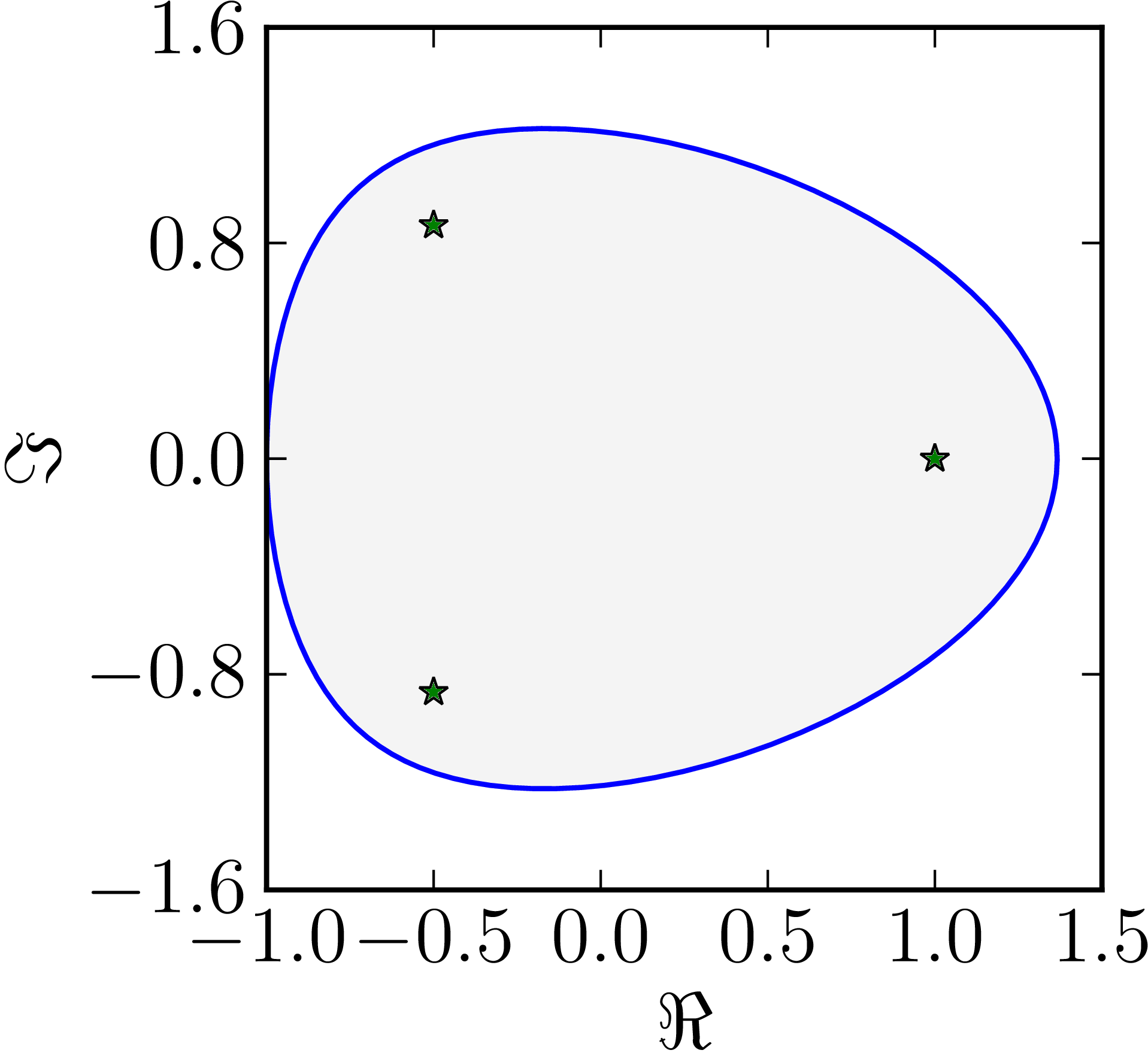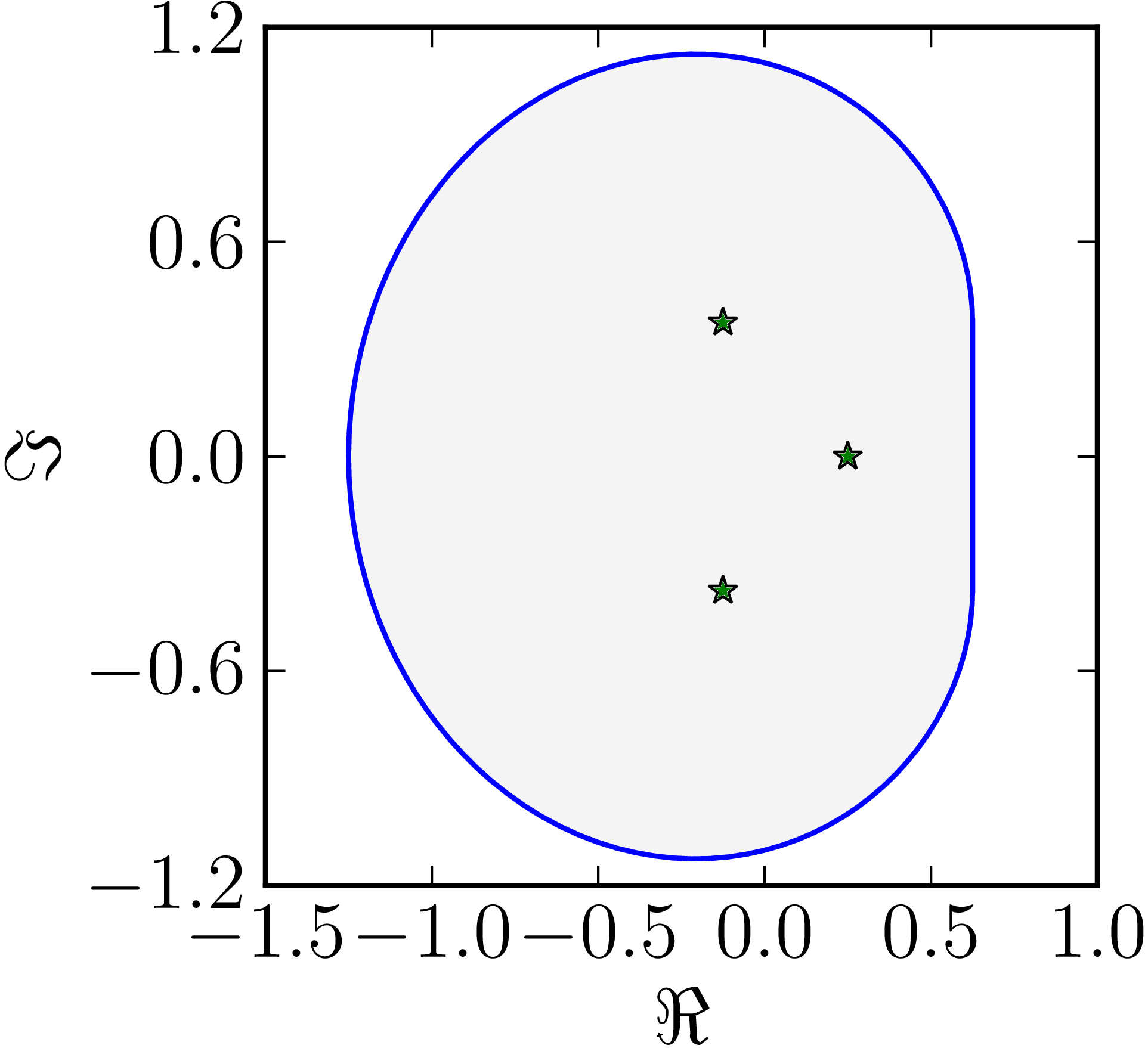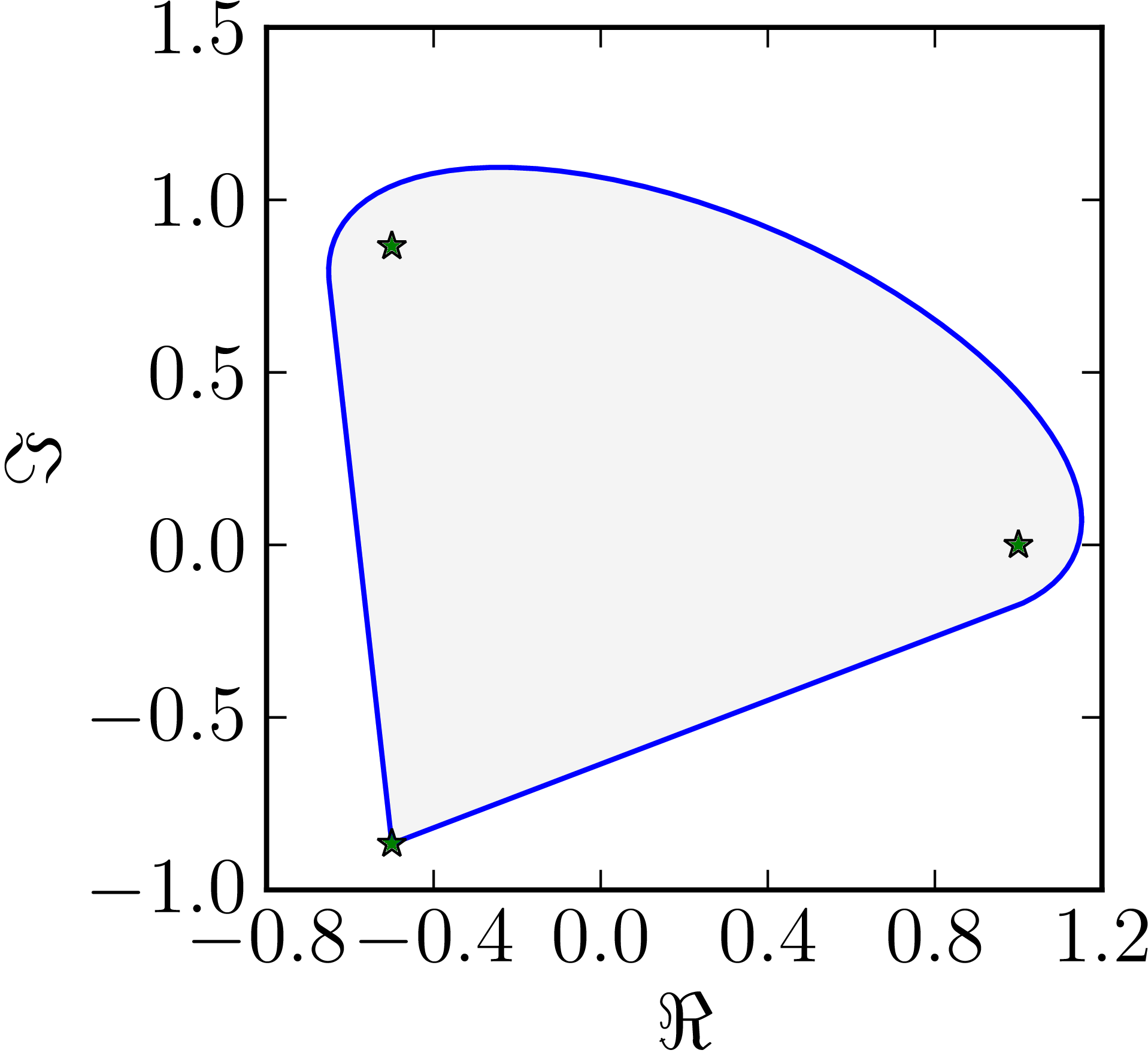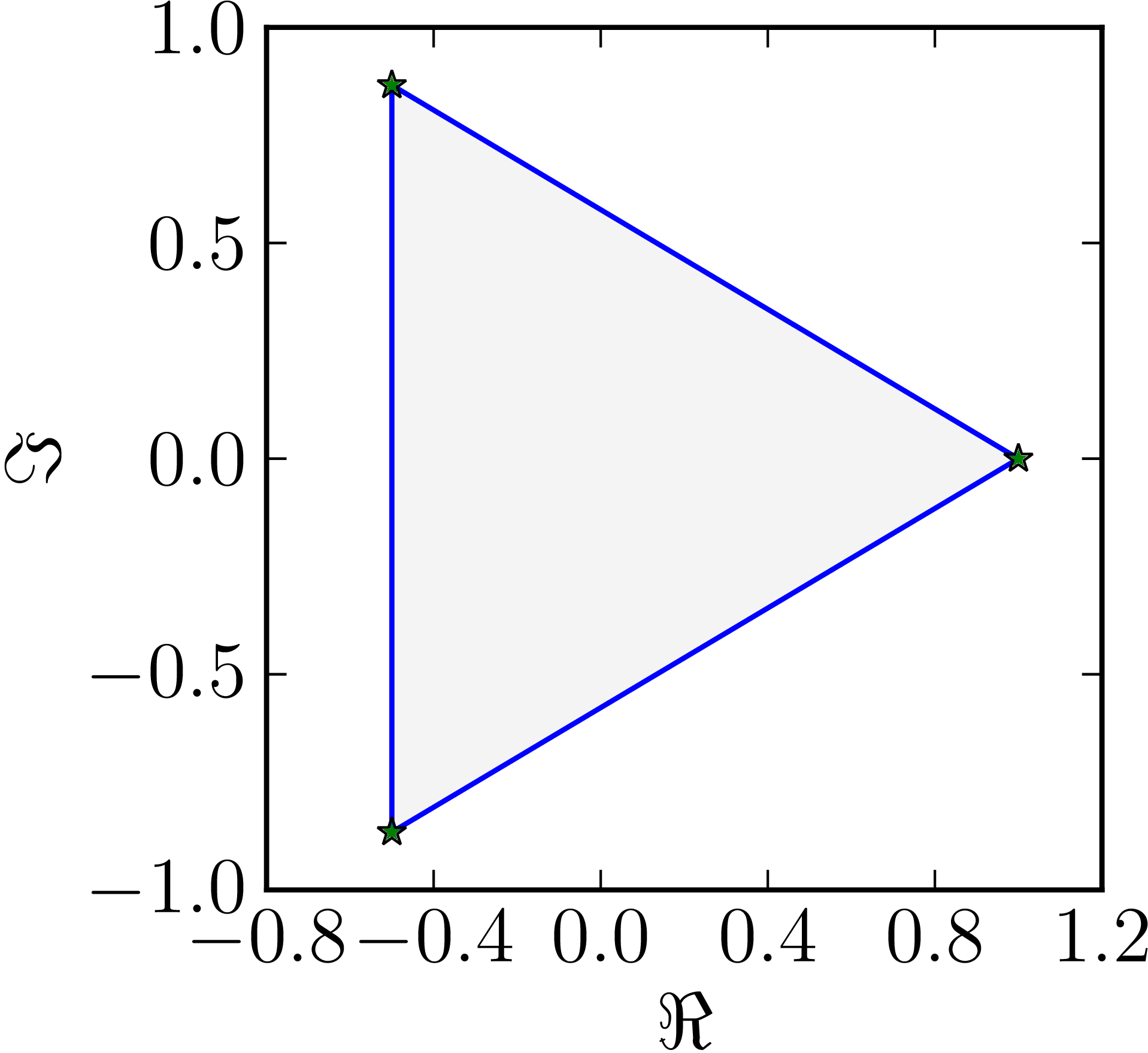In the case of a $3\times 3$ matrix there are four classes of numerical ranges :

• Numerical range with zero line segments (ovular)
• Numerical range with one line segment
• Numerical range with two line segments
• Numerical range with three line segments (triangular)

### Example 1

• The matrix $M=\begin{pmatrix}1&1&1\\0&\ee^{\frac{2\ii}{3}}&1\\0&0&\ee^{\frac{4\ii}{3}}\end{pmatrix}$ has an ovular numerical range
Example of a numerical range whose boundary includes zero line segments.### Example 2

• The matrix $M=$ has a numerical range whose boundary includes one line segment
Example of a numerical range whose boundary includes one line segment.### Example 3

• The matrix $M=\begin{pmatrix}1&1&0\\0&\ee^{\frac{2\ii}{3}}&0\\0&0&\ee^{\frac{4\ii}{3}}\end{pmatrix}$ has a numerical range whose boundary includes two line segments
Example of a numerical range whose boundary includes two line segments.### Example 4

• The matrix $M=\begin{pmatrix}1&0&0\\0&\ee^{\frac{2\ii}{3}}&0\\0&0&\ee^{\frac{4\ii}{3}}\end{pmatrix}$ has a triangular numerical range. Notice that the matrix is normal.
Example of a numerical range whose boundary includes three line segments.1. D. S. Keeler, L. Rodman, and I. M. Spitkovsky, “The numerical range of 3x3 matrices,” Linear Algebra and its Applications, vol. 252, no. 1-3, pp. 115–139, 1997, [Online]. Available at: https://dx.doi.org/10.1016/0024-3795(95)00674-5.